# Chapter 13: Probability Exercise 13.3

In this page we have Class 12 Maths NCERT Solutions Chapter 13: Probability for EXERCISE 13.3 . Hope you like them and do not forget to like , social share and comment at the end of the page.

Question 1
An urn contains 5 red and 5 black balls. A ball is drawn at random, its colour is noted and is returned to the urn. Moreover, 2 additional balls of the colour drawn are put in the urn and then a ball is drawn at random. What is the probability that the second ball is red?
Solution
The urn contains 5 red and 5 black balls.
Let a red ball be drawn in the first attempt.
P (drawing a red ball in First turn) = 5/10 =1/2
If two red balls are added to the urn, then the urn contains 7 red and 5 black balls.
P (drawing a red ball in second turn) = 7/12
Let a black ball be drawn in the first attempt.
P (drawing a black ball in the first attempt) = 5/10 =1/2
If two black balls are added to the urn, then the urn contains 5 red and 7 black balls.
P (drawing a red ball in second turn) = 5/12
So, probability of drawing second ball as red is
= P (drawing a red ball in first turn) P (drawing a red ball in second turn) + P (drawing a black ball in first turn) P (drawing a red ball in second turn)
= ½ × 7/12 + ½ × 5/12 = 1/2

Question 2
A bag contains 4 red and 4 black balls, another bag contains 2 red and 6 black balls. One of the two bags are selected at random and a ball is drawn from the bag which is found to be red. Find the probability that the ball is drawn from the first bag.
Solution
Let X and Y be the events of selecting first bag and second bag respectively.
P(X) =1/2
P(Y) =1/2
Let A be the event of getting a red ball.
P(A|X) = 4/8 =1/2
P(A|Y) = 2/8 =1/4
The probability of drawing a ball from the first bag, given that it is red, is given by P
(Y|A)
By using Bayes’ theorem, we obtain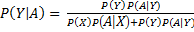Substituting the values given above
P(Y|A) = 2/3

Question 3
Of the students in a college, it is known that 60% reside in hostel and 40% are day scholars (not residing in hostel). Previous year results report that 30% of all students who reside in hostel attain A grade and 20% of day scholars attain A grade in their annual examination. At the end of the year, one student is chosen at random from the college and he has an A grade, what is the probability that the student is hostler?
Solution
Let X and Y be the events that the student is a hostler and a day scholar respectively
and A be the event that the chosen student gets grade A.
P(X) =.60 and P(Y) =.40
P(A|X) = .30
P(A|Y) = .20
The probability that a randomly chosen student is a hostler, given that he has an A
By using Bayes’ theorem, we obtain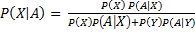Substituting the values given above
=9/13

Question 4:
In answering a question on a multiple-choice test, a student either knows the answer or guesses. Let ¾ be the probability that he knows the answer and ¼ be the probability that he guesses. Assuming that a student who guesses at the answer will be correct with probability ¼  . What is the probability that the student knows the answer given that he answered it correctly?
Solution
Let X and Y be the respective events that the student knows the answer and he
P(X) =1/4 and P(Y) =3/4
Let A be the event that the answer is correct.
The probability that the student answered correctly, given that he knows the answer, is 1.
P (A|X) = 1
Probability that the student answered correctly, given that he guessed, is .
P(A|Y) =1/4
The probability that the student knows the answer, given that he answered it correctly,
is given by P( X|A)
By using Bayes’ theorem, we obtainSubstituting the values given above
=12/13

Question 5
A laboratory blood test is 99% effective in detecting a certain disease when it is in fact, present. However, the test also yields a false positive result for 0.5% of the healthy person tested (that is, if a healthy person is tested, then, with probability 0.005, the test will imply he has the disease). If 0.1 percent of the population actually has the disease, what is the probability that a person has the disease given that his test result is positive?
Solution
Let X and Y be the respective events that a person has a disease and a person has no
disease.
Since X and Y are events complimentary to each other,
P (X) + P (Y) = 1
P (Y) = 1 − P (X) = 1 − 0.001 = 0.999
Let A be the event that the blood test result is positive.
P(A|X) =.99
P(A|Y) = .005
Probability that a person has a disease, given that his test result is positive, is given by
P (X|A).
By using Bayes’ theorem, we obtainSubstituting the values given above
=22/133

Question 6
There are three coins. One is two headed coin (having head on both faces), another is a biased coin that comes up heads 75% of the time and third is an unbiased coin. One of the three coins is chosen at random and tossed, it shows heads, what is the probability that it was the two-headed coin?
Solution
Let X, Y, and Z be the respective events of choosing a two-headed coin, a biased coin, and an unbiased coin.
P(X) =P(Y) =P(Z) =1/3
Let A be the event that the coin shows heads.
P(A|X) =1
Probability of heads coming up, given that it is a biased coin= 75%
P(A|Y) =.75
Since the third coin is unbiased, the probability that it shows heads is always.
P(A|Z) =.5
The probability that the coin is two-headed, given that it shows heads, is given by
P (X|A).
By using Bayes’ theorem, we obtain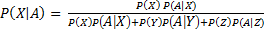Substituting the values given above
=4/9

Question 7
An insurance company insured 2000 scooter drivers, 4000 car drivers and 6000 truck drivers. The probability of accidents is 0.01, 0.03 and 0.15 respectively. One of the insured persons meets with an accident. What is the probability that he is a scooter driver?
Solution
Let X, Y, and Z be the respective events that the driver is a scooter driver, a car
driver, and a truck driver.
There are 2000 scooter drivers, 4000 car drivers, and 6000 truck drivers.
Total number of drivers = 2000 + 4000 + 6000 = 12000
P (X) = P (driver is a scooter driver) = 2000/12000=1/6
P (Y) = P (driver is a car driver) = 4000/12000= 1/3
P (Z) = P (driver is a truck driver) = 6000/12000=1/2
Let A be the event that the person meets with an accident.
P(A|X) =.01= 1/100
P(A|Y) =.03= 3/100
P(A|Z) =.15= 3/20
The probability that the driver is a scooter driver, given that he met with an accident, is
given by P (X|A).
By using Bayes’ theorem, we obtainSubstituting the values given above
=1/52
Question 8
A factory has two machines A and B. Past record shows that machine A produced 60% of the items of output and machine B produced 40% of the items. Further, 2% of the item produced by machine A and 1% produced by machine B were defective. All the items are put into one stockpile and then one item is chosen at random from this and is found to be defective. What is the probability that was produced by machine B?
Solution
Let X and Y be the respective events of items produced by machines A and B.
P(X) = .60=
P(Y) =.40
Let Z be the event that the produced item was found to be defective.
Probability that machine A produced defective items, P (Z|X) = .02
Probability that machine B produced defective items, P (Z|Y) =.01
The probability that the randomly selected item was from machine B, given that it is
defective, is given by P (Y|Z).
By using Bayes’ theorem, we obtain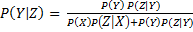Substituting the values given above
=1/4

Question 9
Two groups are competing for the position on the board of directors of a corporation. The probabilities that the first and the second groups will win are 0.6 and 0.4 respectively. Further, if the first group wins, the probability of introducing a new product is 0.7 and the corresponding probability is 0.3 if the second group wins. Find the probability that the new product introduced was by the second group.
Solution
Let X and Y be the respective events that the first group and the second group win the
competition. Let A be the event of introducing a new product.
P (X) = Probability that the first group wins the competition = 0.6
P (Y) = Probability that the second group wins the competition = 0.4
P (A|X) = Probability of introducing a new product if the first group wins = 0.7
P (A|Y) = Probability of introducing a new product if the second group wins = 0.3
The probability that the new product is introduced by the second group is given by
P (Y|A).
By using Bayes’ theorem, we obtainSubstituting the values given above
=2/9

Question 10
Suppose a girl throws a die. If she gets a 5 or 6, she tosses a coin three times and notes the number of heads. If she gets 1, 2, 3 or 4, she tosses a coin once and notes whether a head or tail is obtained. If she obtained exactly one head, what is the probability that she threw 1, 2, 3 or 4 with the die?
Solution
Let X be the event that the outcome on the die is 5 or 6 and Y be the event that the
outcome on the die is 1, 2, 3, or 4.
P(X) =2/6 = 1/3
P(Y) = 4/6 =2/3
Let A be the event of getting exactly one head.
P (A|X) = Probability of getting exactly one head by tossing the coin three times if she
gets 5 or 6
= 3/8 (As exactly one head comes 3 times in 8 total possible outcome HTT, THT, TTH, HHH, TTT, HHT, TTH, HTH)
P (A|Y) = Probability of getting exactly one head in a single throw of coin if she gets 1,
2, 3, or 4
=1/2
The probability that the girl threw 1, 2, 3, or 4 with the die, if she obtained exactly one
head, is given by P (Y|A).
By using Bayes’ theorem, we obtainSubstituting the values given above
=8/11

Question 11
A manufacturer has three machine operators A, B and C. The first operator A produces 1% defective items, whereas the other two operator’s B and C produce 5% and 7% defective items respectively. A is on the job for 50% of the time, B is on the job for 30% of the time and C is on the job for 20% of the time. A defective item is produced, what is the probability that was produced by A?
Solution
Let X, Y, and Z be the respective events of the time consumed by machines A, B, and
C for the job.
P(X) = 50% = .50
P(Y) = 30%= .30
P(Z) = 20% =.20
Let E be the event of producing defective items.
P(E|X) = 1% = .01
P(E|Y) = 5%= .05
P(E|Z) = 7% = .07
The probability that the defective item was produced by A is given by P (X|E).
By using Bayes’ theorem, we obtain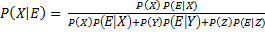Substituting the values given above
=5/34
Question 12
A card from a pack of 52 cards is lost. From the remaining cards of the pack, two cards are drawn and are found to be both diamonds. Find the probability of the lost card being a diamond.
Solution
Let X and Y be the respective events of choosing a diamond card and a card which is
not diamond.
Let A denote the lost card.
Out of 52 cards, 13 cards are diamond and 39 cards are not diamond.
P(X) =13/52 =1/4
P(Y) = 39/52 =3/4
When one diamond card is lost, there are 12 diamond cards out of 51 cards.
Two cards can be drawn out of 12 diamond cards in ways (Favorable outcome) = 12C2
2 cards can be drawn out of 51 cards in ways (Total Outcome) = 51C2.
The probability of getting two cards, when one diamond card is lost, is given by P (A|X).
= 12C2 / 51C2 =222/425
When the lost card is not a diamond, there are 13 diamond cards out of 51 cards.
Two cards can be drawn out of 13 diamond cards in ways (Favorable outcome) = 13C2
2 cards can be drawn out of 51 cards in ways. (Total Outcome) = 51C2.
The probability of getting two cards, when one card is lost which is not diamond, is given
by P (A|Y).
= 13C2 / 51C2 =26/425
The probability that the lost card is diamond is given by P (X|A).
By using Bayes’ theorem, we obtainSubstituting the values given above
=5/34
=11/50
Question 13
Probability that A speaks truth is 4/5. A coin is tossed. A report that a head appears. The
probability that actually there was head is
A. 4/5
B. 1/2
C.1/5
D.2/5
Solution
Let X and Y be the events such that
X: A speaks truth
Y: A speaks false
Let B be the event that a head appears.
P(X) =4/5
P(Y) =1- 4/5 = 1/2
If a coin is tossed, then it may result in either head (H) or tail (T).
The probability of getting a head is 1/2 whether A speaks truth or not.
P(B|X) = 1/2
P(B|Y) = 1/2
The probability that there is actually a head is given by P (X|B).
By using Bayes’ theorem, we obtain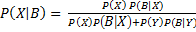Substituting the values given above
=4/5
Therefore, the correct answer is A.
Question 14
If A and B are two events such that A  Ì B and P (B) ≠ 0, then which of the following is correct?
A. P(A|B) = P(B) /P(A)
B. P(A|B) < P(A)
C.  P(A|B) ≥ P(A)
D. None of these
Solution
If A Ì B, then A ∩ B = A
P (A ∩ B) = P (A)
Now
P(A|B) = P (A ∩ B)/P(B)
= P (A)/ P(B) –(1)
Also, P (A) < P (B)
It is known that, P (B) ≤ 1
1/ P (B)   ≥ 1
P(A) /P(B) ≥  P(A)
Now  from (1)
P(A|B) = P (A)/ P(B)
So
P(A|B) ≥  P(A)   -(2)
Thus, from (3), it can be concluded that the relation given in alternative C is correct.

Download Class 12 Maths NCERT Solutions Chapter 13: Probability Exercise 13.3 as pdf

## Related Topics

Go back to Class 12 Main Page using below links